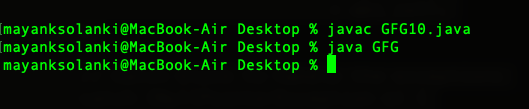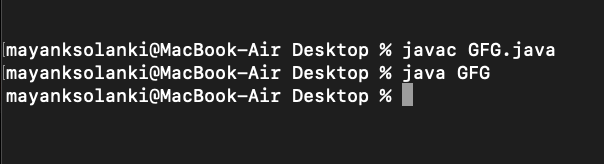Open in App
Not now

# Java.lang.Runtime class in Java

• Difficulty Level : Medium
• Last Updated : 06 May, 2022

In Java, the Runtime class is used to interact with Every Java application that has a single instance of class Runtime that allows the application to interface with the environment in which the application is running. The current runtime can be obtained from the getRuntime() method.

Example 1:

## Java

 `// Java Program to Illustrate Runtime class` `// Via getRuntime(), freeMemory() and` `// totalMemory() Method`   `// Importing required classes` `import` `java.lang.*;` `import` `java.util.*;`   `// Main class` `public` `class` `GFG {`   `    ``// Main driver method` `    ``public` `static` `void` `main(String[] args)` `    ``{`   `        ``// Method 1: getRuntime()` `        ``// Getting the current runtime associated` `        ``// with this process` `        ``Runtime run = Runtime.getRuntime();`   `        ``// Printing the current free memory for this runtime` `        ``// using freeMemory() method` `        ``System.out.println(``""` `+ run.freeMemory());`   `        ``// Method 2: freeMemory()` `        ``// Printing the number of free bytes` `        ``System.out.println(` `            ``""` `+ Runtime.getRuntime().freeMemory());`   `        ``// Method 3: totalMemory()` `        ``// Printing the number of total bytes` `        ``System.out.println(` `            ``""` `+ Runtime.getRuntime().totalMemory());` `    ``}` `}`

Output

```132579840
132285936
134217728```

Example 2:

## Java

 `// Java Program to Illustrate Runtime class` `// Via exec() Method`   `// Importing required classes` `import` `java.util.*;` `import` `java.lang.*;`   `// Main class` `public` `class` `GFG {` `    ``// Main driver method` `    ``public` `static` `void` `main(String[] args)` `    ``{` `        ``// Try block to check for exceptions` `        ``try` `{` `          `  `            ``// Creating a process and execute google-chrome` `            ``Process process = Runtime.getRuntime().exec(` `                ``"google-chrome"``);` `            ``System.out.println(` `                ``"Google Chrome successfully started"``);` `        ``}`   `        ``// Catch block to handle the exceptions` `        ``catch` `(Exception e) {` `            ``// Display the exception on the console` `            ``e.printStackTrace();` `        ``}` `    ``}` `}`

Output:

`Google Chrome successfully started`

Note: Replace with any software you want to start. Here we work on Linux and google-chrome is written like this way only. May differ in windows/mac.

Example 3:

## Java

 `// Java Program to Illustrate Runtime class` `// Via availableProcessors() Method, exit() Method`   `// Importing required classes` `import` `java.util.*;` `import` `java.lang.*;`   `// Main class` `public` `class` `GFG {`   `    ``// Main driver method` `    ``public` `static` `void` `main(String[] args) {`   `        ``// Method 1: availableProcessors()` `        ``// Check the number of processors available` `        ``// and printing alongside` `        ``System.out.println(``""` `+ Runtime.getRuntime()` `                           ``.availableProcessors());`     `        ``// Method 2: exit()` `        ``// Making program to exit` `        ``Runtime.getRuntime().exit(``0``);`   `        ``// Nothing will run now` `        ``System.out.println(``"Program Running Check"``);`   `        ``// Note: here we will notice that there will be no` `        ``// output generated on console`   `    ``}` `}`

Output

`4`

From the above output, it is made clear that exit() method does not let below print statement to execute as “Program Running Check” is not printed on the console. It can be made clear if we comment out the working of availableProcessors() than exit() method output is as follows:Example 4:

## Java

 `// Java Program to illustrate Runtime Class` `// Via loadLibrary(), runFinalization()` `// gc(), maxMemory()`   `// Class` `public` `class` `GFG {`   `    ``// Main driver method` `    ``public` `static` `void` `main(String[] args)` `    ``{`   `        ``// Method 1: loadLibrary()` `        ``// Loading a library that is home/saket/Desktop` `        ``// folder` `        ``Runtime.getRuntime().loadLibrary(` `            ``"/home/saket/Desktop/Library"``);`   `        ``System.out.println(``"Library Loaded Successfully"``);`   `        ``// Method 2: runFinalization()` `        ``// Run the finalization` `        ``Runtime.getRuntime().runFinalization();`   `        ``// Print statement` `        ``System.out.println(``"Finalized"``);`   `        ``// Method 3: gc()` `        ``// Run the garbage collector` `        ``Runtime.getRuntime().gc();`   `        ``System.out.println(``"Running"``);`   `        ``// Method 4: maxMemory()`   `        ``// Printing  the maximum memory` `        ``System.out.println(` `            ``""` `+ Runtime.getRuntime().maxMemory());` `    ``}` `}`

Output:

```Library Loaded Successfully
Finalized
Running
2147483648```

Example 5:

## Java

 `// Java program to illustrate halt()` `// method of Runtime class` `public` `class` `GFG` `{` `    ``public` `static` `void` `main(String[] args)` `    ``{` `        ``// halt this process` `        ``Runtime.getRuntime().halt(``0``);`   `        ``// print a string, just to see if it process is halted` `        ``System.out.println(``"Process is still running."``);` `    ``}` `}`

Output:From above output it is made clear above program compiled  successfully and run. There is no print statement is execute as we have used halt() method which terminates the further execution of operations.

Example 6:

## Java

 `// Java Program to Illustrate exec() ` `// Method of Runtime class`   `// Importing required classes` `import` `java.io.*;` `import` `java.util.*;`   `// Class` `public` `class` `GFG {`   `    ``// Main driver method` `    ``public` `static` `void` `main(String[] args) {`   `        ``// Try block to check for exceptions` `        ``try` `{`   `            ``// Declaring a string array` `            ``String[] cmd = ``new` `String[``2``];`   `            ``// Initializing elements of string array` `            ``cmd[``0``] = ``"atom"``;` `            ``cmd[``1``] = ``"File.java"``;`   `            ``// Creating a file with directory from local systems` `            ``File dir = ``new` `File(``"/Users/mayanksolanki/Desktop"``);`   `            ``// Creating a process by creating Process class object` `            ``// and execute above array using exec() method` `            ``Process process = Runtime.getRuntime().exec(cmd, ``null``);`   `            ``// Display message on console for successful execution` `            ``System.out.println(``"File.java opening."``);` `        ``}`   `        ``// Catch block to handle exceptions` `        ``catch` `(Exception e) {`   `            ``// Display exceptions with line number` `            ``// using printStackTrace() method` `            ``e.printStackTrace();` `        ``}` `    ``}` `}`

Output:

`File.java opening.`